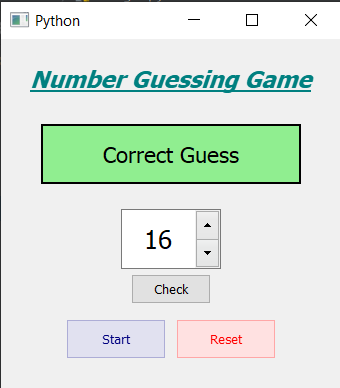Skip to content
Related Articles
PyQt5 – Number Guessing Game
• Last Updated : 03 Jul, 2020

In this article we will see how we can create a number guessing name using PyQt5. The Number guessing game is all about guessing the number randomly chosen by the computer in the given number of chances. Below is how game will look likeGUI implementation steps
1. Create a head label to show the game name, set its alignment font and color
2. Create a info label that gives all the information and set its style sheet
3. Create a spin box to change the guessed number
4. Create a push button to check the guess
5. Create a start and reset buttons and add color effect to them

Back end implementation steps
1. Create a variable number that will store the random number
2. Add action to the start button
3. Inside the start button action get the random number from 1 to 20 using random method
4. Set the text to the info label and change its color to grey
5. Add action to the check button
6. Inside the check button action, get the spin box value and compare it with the random number
7. If numbers are equal change info label color to green and set text as “correct”
8. Else check if smaller then say number is smaller guess again similarly do if number is bigger
9. Add action to the reset button
10. Inside the reset action set welcome text to info label and set its color to grey

Below is the implementation

 `# importing libraries``from` `PyQt5.QtWidgets ``import` `*` `from` `PyQt5 ``import` `QtCore, QtGui``from` `PyQt5.QtGui ``import` `*` `from` `PyQt5.QtCore ``import` `*` `import` `random``import` `sys`` ` ` ` `class` `Window(QMainWindow):`` ` `    ``def` `__init__(``self``):``        ``super``().__init__()`` ` `        ``# setting title``        ``self``.setWindowTitle(``"Python "``)`` ` `        ``# setting geometry``        ``self``.setGeometry(``100``, ``100``, ``340``, ``350``)`` ` `        ``# calling method``        ``self``.UiComponents()`` ` `        ``# showing all the widgets``        ``self``.show()`` ` `        ``# number``        ``self``.number ``=` `0`` ` `    ``# method for components``    ``def` `UiComponents(``self``):`` ` `        ``# creating head label``        ``head ``=` `QLabel(``"Number Guessing Game"``, ``self``)`` ` `        ``# setting geometry to the head``        ``head.setGeometry(``20``, ``10``, ``300``, ``60``)`` ` `        ``# font``        ``font ``=` `QFont(``'Times'``, ``14``)``        ``font.setBold(``True``)``        ``font.setItalic(``True``)``        ``font.setUnderline(``True``)`` ` `        ``# setting font to the head``        ``head.setFont(font)`` ` `        ``# setting alignment of the head``        ``head.setAlignment(Qt.AlignCenter)`` ` `        ``# setting color effect to the head``        ``color ``=` `QGraphicsColorizeEffect(``self``)``        ``color.setColor(Qt.darkCyan)``        ``head.setGraphicsEffect(color)`` ` `        ``# creating a label that will give the info``        ``self``.info ``=` `QLabel(``"Welcome"``, ``self``)`` ` `        ``# setting geometry to the info label``        ``self``.info.setGeometry(``40``, ``85``, ``260``, ``60``)`` ` `        ``# making the info label multi line``        ``self``.info.setWordWrap(``True``)`` ` `        ``# setting font and alignment``        ``self``.info.setFont(QFont(``'Times'``, ``13``))``        ``self``.info.setAlignment(Qt.AlignCenter)`` ` `        ``# setting style sheet``        ``self``.info.setStyleSheet(``"QLabel"``                                ``"{"``                                ``"border : 2px solid black;"``                                ``"background : lightgrey;"``                                ``"}"``)`` ` `        ``# creating a spin box to set the number``        ``self``.spin ``=` `QSpinBox(``self``)`` ` `        ``# setting range to the the spin box``        ``self``.spin.setRange(``1``, ``20``)`` ` `        ``# setting geometry to the spin box``        ``self``.spin.setGeometry(``120``, ``170``, ``100``, ``60``)`` ` `        ``# setting alignment and font``        ``self``.spin.setAlignment(Qt.AlignCenter)``        ``self``.spin.setFont(QFont(``'Times'``, ``15``))`` ` `        ``# creating a push button to check the guess number``        ``check ``=` `QPushButton(``"Check"``, ``self``)`` ` `        ``# setting geometry to the push button``        ``check.setGeometry(``130``, ``235``, ``80``, ``30``)`` ` `        ``# adding action to the check button``        ``check.clicked.connect(``self``.check_action)`` ` `        ``# creating a start button``        ``start ``=` `QPushButton(``"Start"``, ``self``)``        ``start.setGeometry(``65``, ``280``, ``100``, ``40``)`` ` `        ``# reset button to reset the game``        ``reset_game ``=` `QPushButton(``"Reset"``, ``self``)`` ` `        ``# setting geometry to the push button``        ``reset_game.setGeometry(``175``, ``280``, ``100``, ``40``)`` ` `        ``# setting color effect``        ``color_red ``=` `QGraphicsColorizeEffect()``        ``color_red.setColor(Qt.red)``        ``reset_game.setGraphicsEffect(color_red)`` ` `        ``color_green ``=` `QGraphicsColorizeEffect()``        ``color_green.setColor(Qt.darkBlue)``        ``start.setGraphicsEffect(color_green)`` ` `        ``# adding action to the  button``        ``start.clicked.connect(``self``.start_action)``        ``reset_game.clicked.connect(``self``.reset_action)`` ` `    ``def` `start_action(``self``):`` ` `        ``# making label green``        ``self``.info.setStyleSheet(``"QLabel"``                                ``"{"``                                ``"border : 2px solid black;"``                                ``"background : lightgrey;"``                                ``"}"``)`` ` `        ``# creating random number``        ``self``.number ``=` `random.randint(``1``, ``20``)`` ` `        ``# setting text to the info label``        ``self``.info.setText(``"Try to guess number between 1 to 20"``)`` ` ` ` `    ``def` `check_action(``self``):`` ` `        ``# get the spin box number``        ``user_number ``=` `self``.spin.value()`` ` `        ``# check the value``        ``if` `user_number ``=``=` `self``.number:`` ` `            ``# setting text to the info label``            ``self``.info.setText(``"Correct Guess"``)``            ``# making label green``            ``self``.info.setStyleSheet(``"QLabel"``                                    ``"{"``                                    ``"border : 2px solid black;"``                                    ``"background : lightgreen;"``                                    ``"}"``)`` ` `        ``elif` `user_number < ``self``.number:`` ` `            ``# giving hint``            ``self``.info.setText(``"Your number is smaller"``)`` ` `        ``else``:`` ` `            ``# giving hint``            ``self``.info.setText(``"Your number is bigger"``)`` ` ` ` `    ``def` `reset_action(``self``):``        ``# making label green``        ``self``.info.setStyleSheet(``"QLabel"``                                ``"{"``                                ``"border : 2px solid black;"``                                ``"background : lightgrey;"``                                ``"}"``)`` ` `        ``# setting text to the info label``        ``self``.info.setText(``"Welcome"``)`` ` ` ` ` ` ` ` ` ` `# create pyqt5 app``App ``=` `QApplication(sys.argv)`` ` `# create the instance of our Window``window ``=` `Window()`` ` `# start the app``sys.exit(App.``exec``())`

Output :

Attention geek! Strengthen your foundations with the Python Programming Foundation Course and learn the basics.

To begin with, your interview preparations Enhance your Data Structures concepts with the Python DS Course. And to begin with your Machine Learning Journey, join the Machine Learning – Basic Level Course

My Personal Notes arrow_drop_up